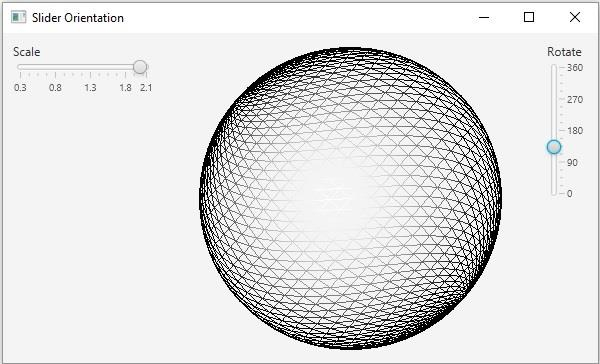# How to change the orientation of a slider in JavaFX?

JavaFX provides a class known as Slider, this represents a slider component that displays a continuous range of values. This contains a track on which the number values are displayed. Along the track, there is a thumb pointing to the numbers. You can provide the maximum, minimum, and initial values of the slider.

In JavaFx you can create a slider by instantiating the javafx.scene.control.Slider class.

## Changing the orientation of a slider

The JavaFX slider can be either vertical or horizontal by default on instantiating the Slider class a horizontal slider is created. The orientation property specifies the orientation of the current slider i.e. horizontal or vertical. You can set the value to this property using the setOrientation() method.

To this method you can pass one of the following two constants as a parameter −

• Orientation.VERTICAL

• Orientation.HORIZONTAL

Therefore, to change the orientation of the slider you need to invoke the setOrientation method bypassing one of above two values as a parameter.

## Example

import javafx.application.Application;
import javafx.beans.value.ChangeListener;
import javafx.beans.value.ObservableValue;
import javafx.geometry.Insets;
import javafx.geometry.Orientation;
import javafx.scene.Scene;
import javafx.scene.control.Label;
import javafx.scene.control.Slider;
import javafx.scene.layout.BorderPane;
import javafx.scene.layout.VBox;
import javafx.scene.shape.DrawMode;
import javafx.scene.shape.Sphere;
import javafx.scene.transform.Rotate;
import javafx.scene.transform.Scale;
import javafx.stage.Stage;
public class SliderOrientation extends Application {
public void start(Stage stage) {
//Drawing a Sphere
Sphere sphere = new Sphere();
sphere.setDrawMode(DrawMode.LINE);
//Creating a slider for rotation
Slider slider1 = new Slider(0, 360, 0);
//Setting its orientation to vertical
slider1.setOrientation(Orientation.VERTICAL);
slider1.setShowTickLabels(true);
slider1.setShowTickMarks(true);
slider1.setMajorTickUnit(90);
slider1.setBlockIncrement(10);
Rotate rotate = new Rotate();
public void changed(ObservableValue <?extends Number>observable, Number oldValue, Number newValue){
//Setting the angle for the rotation
rotate.setAngle((double) newValue);
}
});
//Creating a slider for scaling
Slider slider2 = new Slider(0.3, 2.1, 0.6);
//Setting its orientation to Horizontal
slider2.setOrientation(Orientation.HORIZONTAL);
slider2.setShowTickLabels(true);
slider2.setShowTickMarks(true);
slider2.setMajorTickUnit(0.5);
slider2.setBlockIncrement(0.1);
Scale scale = new Scale();
public void changed(ObservableValue <?extends Number>observable, Number oldValue, Number newValue){
scale.setX((double) newValue);
scale.setY((double) newValue);
}
});
//Adding all the transformations to the node
//Creating the pane
BorderPane pane = new BorderPane();
pane.setRight(new VBox(new Label("Rotate"), slider1));
pane.setCenter(sphere);
pane.setLeft(new VBox(new Label("Scale"), slider2));
//Preparing the scene
Scene scene = new Scene(pane, 595, 330);
stage.setTitle("Slider Orientation");
stage.setScene(scene);
stage.show();
}
public static void main(String args[]){
launch(args);
}
}

## Output